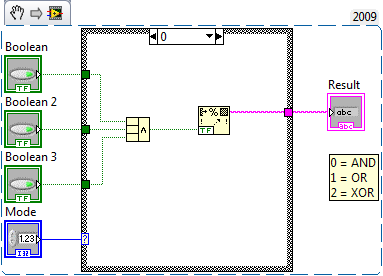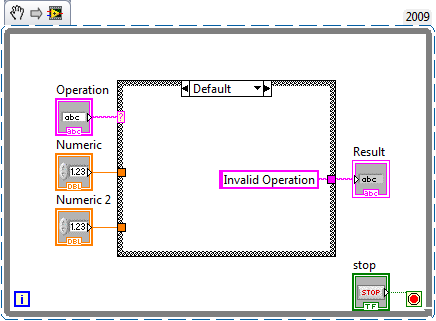# Curriculum and Labs for Engineering Education

cancel
Showing results for
Did you mean:

## Unit 3 - Case Structures: Lesson 3

Introduction

In this lesson we talk about case structures with numeric controls.

Lesson Video

Example SnippetPractice Problem

Procedure: Create the required VI(s) to achieve the following tasks.

Requirements:

1. Create a basic calculator function with the ability to add, subtract, multiply, and divide two numbers and display the output
2. Allow the program to keep on running until a stop button is pressed.

Practice SolutionNext Step

Unit 4 - DAQ: Lesson 1